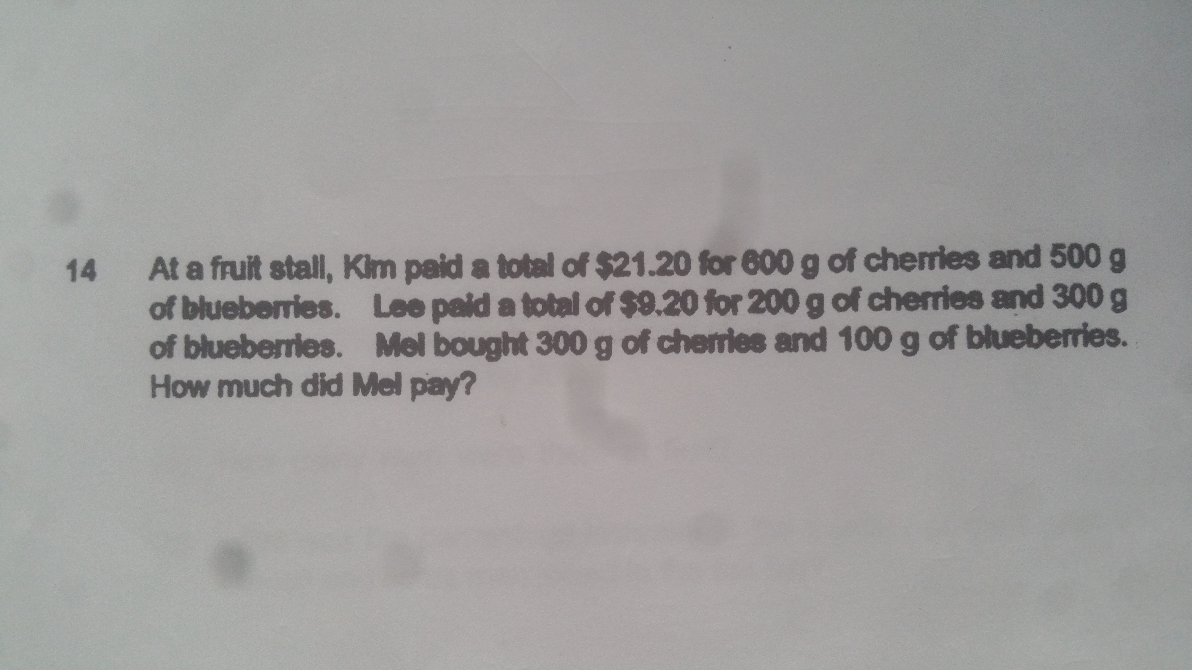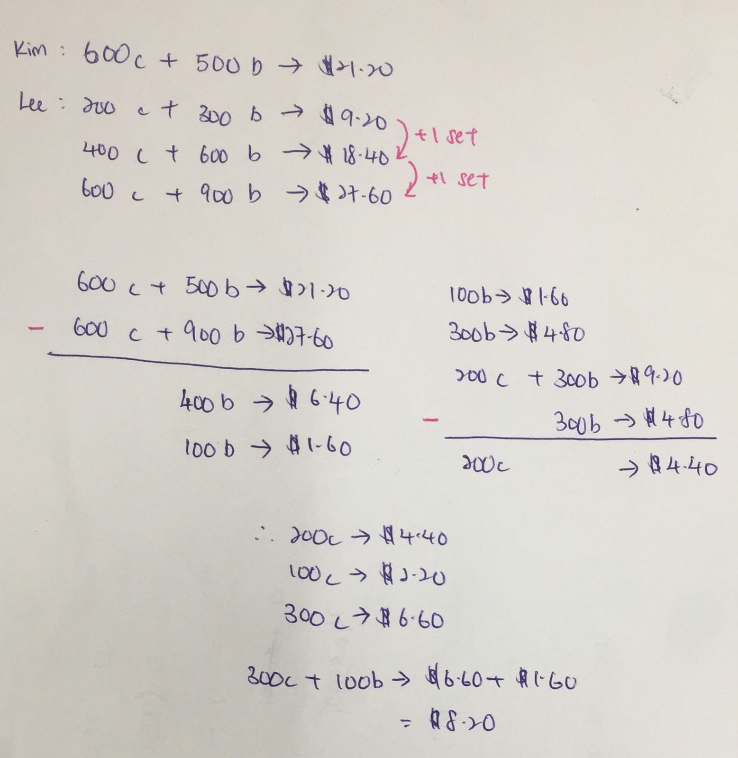# Question0 Replies 0 Likes ✔Accepted Answer

Let C be Cherries, and B be Blueberries

C                         B                       Price

600        +          500         =        \$21.20

200        +          300         =        \$9.20

Therefore, 600C + 500 B – 3(200C +300B) = 21.2 – 3(9.2)

600C +500B – 600C – 900B = 21.2 – 27.6

-400B = -6.4

400B/4 = 6.4/4

100B = 1.6

600C + 5(1.6) = 21.2

600C + 8 = 21.2

600C/6 = 13.2/6

100C = 2.2

300C + 100B = 3(2.2) + 1.6 = 8.4

Mel paid \$8.40.

0 Replies 0 Likes